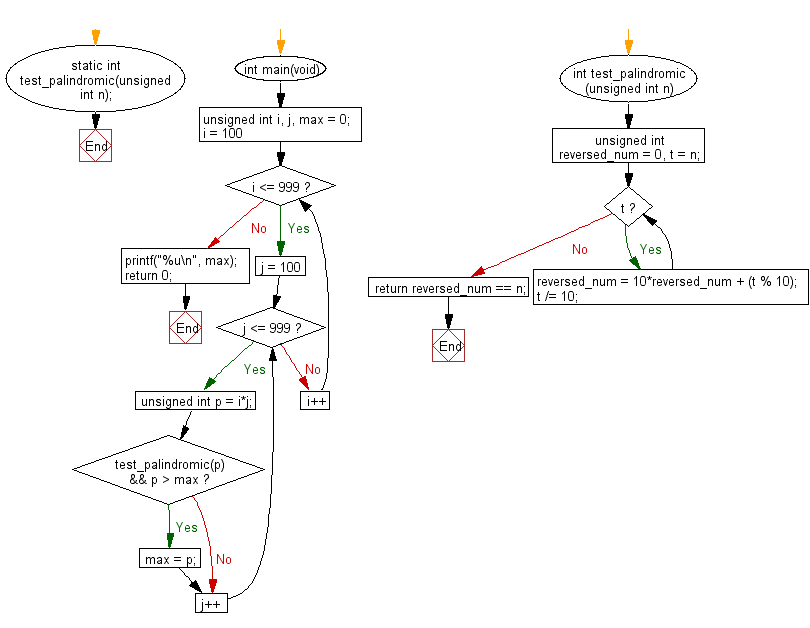﻿ C Program: Largest palindrome from two 3-digit numbers# C Exercises: Find the largest palindrome made from the product of two 3-digit numbers

## C Programming Practice: Exercise-20 with Solution

A palindrome is a word, number, phrase, or other sequence of characters which reads the same backward as forward, such as taco cat or madam or racecar or the number 10801. The largest palindrome made from the product of two 2-digit numbers is 9009 = 91 × 99.
Write a C programming to find the largest palindrome made from the product of two 3-digit numbers.

C Code:

``````#include <stdio.h>
static int test_palindromic(unsigned int n);
int main(void)
{
unsigned int i, j, max = 0;
for (i = 100; i <= 999; i++) {
for (j = 100; j <= 999; j++) {
unsigned int p = i*j;
if (test_palindromic(p) && p > max) {
max = p;
}
}
}
printf("%u\n", max);
return 0;
}
int test_palindromic(unsigned int n)
{
unsigned int reversed_num = 0, t = n;
while (t) {
reversed_num = 10*reversed_num + (t % 10);
t /= 10;
}
return reversed_num == n;
}
``````

Sample Output:

```906609
```

Flowchart:## C Programming Code Editor:

What is the difficulty level of this exercise?

Test your Programming skills with w3resource's quiz.

﻿

## C Programming: Tips of the Day

Maximum value of int:

In C:

```#include <limits.h>
then use
int imin = INT_MIN; // minimum value
int imax = INT_MAX;```

or

```#include <float.h>

float fmin = FLT_MIN;  // minimum positive value
double dmin = DBL_MIN; // minimum positive value

float fmax = FLT_MAX;
double dmax = DBL_MAX;```

Ref : https://bit.ly/3fi8yk9